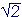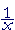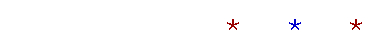Topics in

P R E C A L C U L U S

6

# THE VOCABULARY OFPOLYNOMIAL FUNCTIONS

Terms and factors

FUNCTIONS CAN BE CATEGORIZED, and the simplest type is a polynomial.  We will define it below.  We begin with vocabulary.

1.   When numbers are added or subtracted, they are called terms.  This --

4x2 + 7x − 8

-- is a sum of three terms.  (In algebra we speak of a "sum," even though a term may be subtracted.)

When numbers are multiplied, they are called factors.  This --

1.    (x + 1)(x + 2)(x + 3)

-- is a product of three factors.

2.   A variable is a symbol that takes on different values.  A value is a number.

Thus if x is a variable and we give it the value 4, then 5x + 1 has the value 21.

3.   A constant is a symbol that has a single value.  The symbols 2, 5,,are constants.  When we write

y = ax2 + bx + c,

then  a, b, c  are arbitrary constants to which we may assign a definite value; for example,

y = 5x2 − 2x + 1.

We typically use the beginning letters of the alphabet to denote such constants. We use the letters x, y, z to denote variables.

4.   A monomial in x is a single term of the form  axn,  where a is a
real number and n is a whole number.

The following are monomials in x:

5x3    −6.3x     2

We may say that the number 2 is a monomial in x because 2 = 2x0 = 2·1. (Lesson 21 of Algebra.)

5.   A polynomial in x is a sum of monomials in x.

Example 1.   5x3 − 4x2 + 7x − 8.

The variable, in this case x, is also called the argument of the polynomial.  Here is a polynomial with argument t :

t 2 −5t + 1.

When we write a polynomial, the style is to begin with the highest exponent and go to the lowest.  4, 3, 2, 1.

(For the general form of a polynomial, see Problem 6 below.)

6.   The degree of a term is the sum of the exponents of all the variables in that term.

In functions of a single variable, such as x, the degree of a term is simply the exponent.

Example 2.  The term  5x3  is of degree 3 in the variable x.

Example 3.  This term  2xy2z3 is of degree 1 + 2 + 3 = 6 in the variables x, y, and z

Example 4.  Here are all possible terms of the 4th degree in the variables x and y:

x4,  x3y,  x2y2,  xy3,  y4.

In each term, the sum of the exponents is 4.  As the exponent of x decreases, the exponent of y increases.

Problem 1.   Write all possible terms of the 5th degree in the variables x and y.

x5,  x4y,  x3y2,   x2y3,  xy4,  y5.

7.   The leading term of a polynomial is the term of highest degree.

Example 5.  The leading term of this polynomial  5x3 − 4x2 + 7x − 8  is 5x3.

8.   The leading coefficient of a polynomial is the coefficient of the leading term.

Example 6.  The leading coefficient of that polynomial  is 5.

9.   The degree of a polynomial is the degree of the leading term.

Example 7.  The degree of this polynomial  5x3 − 4x2 + 7x − 8   is 3.

Here is a polynomial of the first degree:  x − 2.

1 is the highest exponent.

10.   The constant term of a polynomial is the term of degree 0; it is the term in which the variable does not appear.

Example 8.  The constant term of this polynomia --

5x3 − 4x2 + 7x − 8,

-- is −8.

The constant term of this polynomial --

ax3 + bx2 + cx + d

-- is d.

Problem 2.   Which of the following is a polynomial?  If an expression is a polynomial, name its degree, and say the variable that the polynomial is in.

a)  x3 − 2x2 − 3x − 4    Polynomial of the 3rd degree in x.

b)  3y2 + 2y + 1    Polynomial of the 2nd degree in y.

c)  x3 + 2+ 1     This is not a polynomial, becauseis not a
whole number power. It is x½.

d)  z + 2  Polynomial of the first degree in z.

e)  x2 − 2xNot a polynomial, because= x−1, which is not a whole number power.

Problem 3.    Name the degree, the leading coefficient, and the constant term.

a)  f(x) = 6x3 + 7x2 − 3x + 1

3rd degree.  Leading coefficient, 6.  Constant term, 1.

b)  g(x) = −x + 2

1st degree.  Leading coefficient, −1.  Constant term, 2.

c)  h(x) = 4x5

5th degree.  Leading coefficient, 4.  Constant term, 0.

d)  f(h) = h2 − 7h − 5

2nd degree.  Leading coefficient, 1.  Constant term, −5.

Example 9.   Name the degree, the leading coefficient, and the constant term:

(5x + 1)(3x − 1)(2x + 5)3.

If we were to multiply out, then the degree of the product would be the sum of the degrees of each factor:  1 + 1 + 3 = 5.   For,

(5x + 1)(3x − 1)(2x + 5)3 = (5x + 1)(3x − 1)(8x3 + . . . + 53).

The leading coefficient would be the product of all the leading coefficients:  5· 3· 8 = 15· 8 = 120.

And the constant term would be the product of all the constant terms:  1· (−1)· 53 = −1· 125 = −125.

Problem 4.   Name the degree, the leading coefficient, and the constant term.

a)  f(x) = (x − 1)(x2 + x − 6)

Degree: 3.  Leading coefficient: 1.  Constant term: 6.

b)  g(x) = (x + 2)2(x − 3)3(2x + 1)4

Degree: 9.  Leading coefficient: 12· 13· 24 = 16.
Constant term:  22· (-3)3· 14 = 4· (−27) = −108

c)  f(x) = (2x + 1)5

Degree: 5.  Leading coefficient: 25 = 32.  Constant term: 15 = 1.

d)  h(x) = x(x − 2)5(x + 3)2

Degree: 8.  Leading coefficient: 1.  Constant term: 0.

11.   The general form of a polynomial shows the terms of all possible degree.  Here, for example, is the general form of a polynomial of the third degree:

ax3 + bx2 + cx + d

Notice that there are four constants: a, b, c, d.

In the general form, the number of constants, because of the term of degree 0, is always one more than the degree of the polynomial.

Now, to indicate a polynomial of the 50th degree, we cannot indicate the constants by resorting to different letters.  Instead, we use sub-script notation.  We use one letter, such as a, and indicate different constants by means of sub-scripts.  Thus, a1 ("a sub-1") will be one constant.  a2 ("a sub-2") will be another.  And so on.  Here, then, is the general form of a polynomial of the 50th degree:

a50x50 + a49x49 + . . . + a2x2 + a1x + a0

The constant ak -- for each sub-script k  (k = 0, 1, 2, . . . , 50) -- is the coefficient of xk.

Notice that there are 51 constants.  The constant term a0 is the 51st.

Problem 5.

a)  Using subscript notation, write the general form of a polynomial of
a)  the fifth degree in x.

a5x5 + a4x4 + a3x3 + a2x2 + a1x + a0

b)  In that general form, how many constants are there?  6

c)  Name the six constants of this fifth degree polynomial:

x5 + 6x2x.

a5 = 1.  a4 = 0.  a3 = 0.  a2 = 6.  a1 = −1.  a0 = 0.

Problem 6.

a)  Indicate the general form of a polynomial in x of degree n.

anxn + an−1xn−1 + . . . + a1x +a0

n is a whole number, the a's are real numbers, and an0.

b)  A polynomial of degree n has how many constants?  n + 1

12.  A polynomial function has the form

y = A polynomial

A polynomial function of the first degree, such as  y = 2x + 1,  is called a linear function; while a polynomial function of the second degree, such as  y = x2 + 3x − 2,  is called a quadratic.

Domain and range

The natural domain of any polynomial function is< x <.

x may take on any real value.  Consider the graphs of y = x2 , and y = x3.

Problem 7.   Let f(x) be the function with the given, restricted domain.  Describe its range.

a)  f(x) = x2,   −3 x 3

The problem means: When x takes values restrticted to that interval, what is the lowest value that y will have? What is the highest?

Consider the graph.

0 y 9.  y goes from a low of 0 (at x = 0) to a high of 9 (at both −3 and 3).

b)  f(x) = x3,    −3 x 3

Consider the graph.

−27 y 27.  y goes from a low of −27 (at x = −3) to a high of 27 (at x = 3).

c)  f(x) = x4,   −2 x 1

0 y 16.   y goes from a low of 0, at x = 0, to a high of 16, at x = −2.

x4 is very much like x2.  The exponent is even.

d)  f(x) = x5,   −2 x 1

−32 y 1.   y goes from a low of −32, at x = −2, to a high of 1, at x = 1.

x5 is very much like x3.  The exponent is odd.

In the following Topics we will focus on the graphs of these polynomial functions.Next Topic:  The roots, or zeros, of a polynomial

Please make a donation to keep TheMathPage online.
Even \$1 will help.